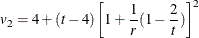### Multivariate Inferences

Multivariate inference based on Wald tests can be done with m imputed data sets. The approach is a generalization of the approach taken in the univariate case (Rubin 1987, p. 137; Schafer 1997, p. 113). Suppose that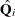and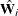are the point and covariance matrix estimates for a p-dimensional parameter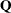(such as a multivariate mean) from the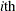imputed data set, i = 1, 2, …, m. Then the combined point estimate forfrom the multiple imputation is the average of the m complete-data estimates: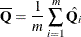Suppose that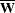is the within-imputation covariance matrix, which is the average of the m complete-data estimates: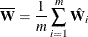And suppose that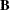is the between-imputation covariance matrix: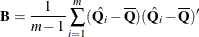Then the covariance matrix associated with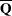is the total covariance matrix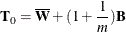The natural multivariate extension of the t statistic used in the univariate case is the F statistic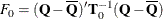with degrees of freedom p and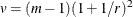where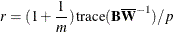is an average relative increase in variance due to nonresponse (Rubin 1987, p. 137; Schafer 1997, p. 114).

However, the reference distribution of the statistic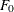is not easily derived. Especially for small m, the between-imputation covariance matrixis unstable and does not have full rank for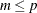(Schafer, 1997, p. 113).

One solution is to make an additional assumption that the population between-imputation and within-imputation covariance matrices are proportional to each other (Schafer, 1997, p. 113). This assumption implies that the fractions of missing information for all components ofare equal. Under this assumption, a more stable estimate of the total covariance matrix is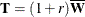With the total covariance matrix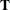, the F statistic (Rubin, 1987, p. 137)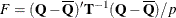has an F distribution with degrees of freedom p and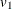, where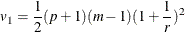For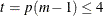, PROC MIANALYZE uses the degrees of freedomin the analysis. For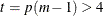, PROC MIANALYZE uses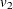, a better approximation of the degrees of freedom given by Li, Raghunathan, and Rubin (1991):